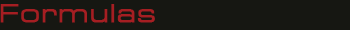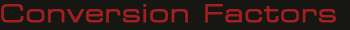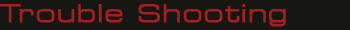# Cylinder Pressure

(PSI) = Force (pounds)/Area (sq. Inch)

# Cylinder Area

(sq. inch) = 3.1416 x Radius Squared

# Cylinder Force

(pounds) = Pressure (PSI) x Area (sq. inch)

# Cylinder Volume/Gallons

(Vol) = (Area x Stroke in inches) / 231

# Fluid Motor Torque

(in. lbs.) T = Pressure (psi) x disp. (cu. in. / rev.) / 6.2822

# Fluid Motor Speed

(RPM) = (231 x GPM) / disp. (cu. in.)

# Fluid Motor Horsepower

= Torque (in. lbs.) x RPM / 63025

# Pump Output Flow

(GPM) = ((RPM) x disp. (cu. in.)) / 231

# Pump Input Horsepower

= GPM x Pressure (psi) / 1714 x eff.

# Pump Input Torque

(T) = (GPM x PSI x 3.06) / RPM /eff

# Pump Displacement Cu. In.

(Disp.) = GPM x 231) / RPM /eff

# Pump Input Speed

( RPM) = (GPM x 231) / Disp. / eff

# Pump Efficiency

(eff) = Actual Flow (GPM) / Theoretical Flow (GPM)

# Pump Flow with PTO Drive

(GPM) = (Engine RPM x % PTO x Disp. x eff) / 231

# Velocity of Oil Flow in Pipe

(Vel) = GPM x 0.3208 / Area• 1 BAR = 14.50 PSI
• 1 Centimeter = 0.3937 Inches
• 1 Cubic Centimeter = 0.06 Cubic Inches
• 1 Cubic Inch = 16.39 Cubic Centimeters
• 1 Gallon = 3.79 Liters
• 1 Gallon = 231 Cubic Inches
• 1 Gallon Per Minute = 3.79 LPM
• 1 HP/ Hr = 2,547 BTU
• 1 Inch = 2.540 Centimeters
• 1 Inch = 25.4 Millimeters
• 1 KW = 1.341 HP
• 1 Liter = 0.26 Gallons
• 1 Liter Per Minute = 0.26 GPM
• 1 Millimeter = 0.039 Inches
• 1 MPA = 145.2 PSI
• 1 PSI = 0.069 BAR# Pump Unusually Noisy

• Low fluid level
• Fluid too heavy ( incorrect viscosity )
• Fluid temperature too low
• Suction line too small
• Restriction or partially clogged suction line
• Suction strainer / filter contaminated
• Suction strainer / filter too small
• Pump running too fast
• Reservoir air-vent plugged
• Air bubbles in fluid
• Air leak at pump inlet plumbing
• Flexible coupling misalignment
• Pump excessively worn

# Pump Takes Too Long or Fails to Respond

• Low fluid level
• Fluid too heavy ( incorrect viscosity )
• Suction strainer / filter contaminated
• Suction strainer / filter too small
• Suction ball valve closed or partially closed
• Reservoir air-vent plugged
• Air leak at pump inlet plumbing
• Pump running too slowly
• Pump failure

# No Pressure in System

• Pump not delivering fluid
• Relief valve mal-adjusted or failed ‘open’
• Internal leakage in control valves, motors or cylinders
• External leakage of component(s)

# Low or Erratic Pressure in System

• Relief valve setting too low
• Relief valve worn or sticking
• Low fluid level
• Fluid too heavy ( incorrect viscosity )
• Air bubbles in fluid
• Control valves not opening / closing fully
• Internal leakage in control valves, motors or cylinders
• External leakage of component(s)
• Pump compensator setting too low
• Pump excessively worn

# Slow or No Actuator Operation

• Pump not delivering correct amount of fluid
• Directional Control not shifting
a. Electrical failure of solenoid, limit switches, etc
b. Insufficient pilot-pressure
c. Interlock device not actuated
• Worn or damaged cylinder or hydraulic motor